## General Question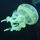# What mathematical equation do I use relating to normal approximation to the binomial?

Asked by peachfuzz (5) April 28th, 2010

I need to figure out what equation to start with to figure out this statistics problem. The question has multiple parts to it but I think if I can just figure out what equation to start with in parts a and b then I can figure out the rest for c, d, e and get the answers on my own.

a. How many votes did each candidate get?

b. If a total of 100 other votes weren’t counted, what is the smallest number of these votes that S would need to have won?

c. If P(S) = 50% what is the binomial probability that she got this minimum amount of votes.

Observing members: 0Composing members: 0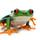@peachfuzz I see you are new here so I will try to explain this without getting into a fight with you. I see that has happened already with another member. No need to call names from either of us.

On Fluther members will try to help you if they can and won’t give you an answer to a homework question or quiz. In statistics, the equation is part of the answer. You already know that if you know the equation, you can plug in the numbers and get the answer. We won’t give you the equation. If you look at the Part A of your question, you can get the answer without even knowing the equation. Once you have the answer you will be able to work backward to get the equation for your teacher.

I know this sounds complicated, but it isn’t. It may take a lot of thought. Do you know the answer to part A? If so, we can go from there. Good luck.

missingbite (7531)“Great Answer” (4) Flag as…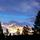@missingbite Well said. I was about to answer, but you said it far better than I could have.

bobbinhood (5896)“Great Answer” (0) Flag as…@bobbinhood Thank you.

missingbite (7531)“Great Answer” (0) Flag as…J = (10000/2)+9 = 5000 + 9 = 5009
S = (10000/2)-9 = 5000 – 9 = 4991

IFF thats right… either way idk how to proceed from there

for (b) I was thinking that S needs 60 but again, don’t know the equation or any way to prove or disprove that. I’m not sure if I’m doing any of this right which is why I asked for help

peachfuzz (5)“Great Answer” (0) Flag as…Is this true? 5009 – 4991 = 9

If not, then you have not given J 9 more votes than S. Try making a single equation using algebra. If the number of votes that S recieves is x, then how many votes did J receive? Use this to make an equation and solve for x.

bobbinhood (5896)“Great Answer” (1) Flag as…@bobbinhood You said that better than I could! Thank you again.

missingbite (7531)“Great Answer” (0) Flag as…@missingbite It appears we make a good tag-team! :)

bobbinhood (5896)“Great Answer” (0) Flag as…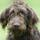@missingbite Kudos

Kayak8 (16452)“Great Answer” (0) Flag as…@bobbinhood Same to you!

Kayak8 (16452)“Great Answer” (0) Flag as…If J-x=9, then J=x+9; J+x = 10000 so x=10000-J; thus J=(10000-J)+9

Am I on the right track? Its been years since I took any math classes… do I multiply or divide or what to simplify that farther to get actually isolate and get the value of J?

peachfuzz (5)“Great Answer” (0) Flag as…You definitely made that more confusing than it had to be, but that does work. You have already shown that you know how to get one J to the other side of the equation. Use the same idea as when you went from J + x = 10,000 to x = 10,000 – J.

bobbinhood (5896)“Great Answer” (0) Flag as…@peachfuzz I agree again with @bobbinhood that you made that a lot harder but also showed me a new way. Good job. Now you are thinking and that is what makes this fun. I will now watch to see you finish the problem.

@Kayak8 Thanks. I think @bobbinhood is a lot smarter than I am. I haven’t looked at stats since flight school. A long time ago.

missingbite (7531)“Great Answer” (0) Flag as…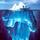@peachfuzz: Here’s an old math classic: A bat and a ball together cost \$1.10. The bat costs a dollar more than the ball. How much does the ball cost? The answer is a nickel—\$1.05 and \$0.05. If X is the cost of the ball in dollars, then the equation is:

(X + 1) + X = 1.10
where (X+1) represents the cost of the bat and X represents the cost of the ball. Your election problem is similar in asking for numbers whose sum and a difference are given.

J defeats S by 9 votes means that S = J+9 or, if you like, J = S-9. (You MUST see why before going on.) The total number of votes is J+S = 10,000. (Ditto) The solution to (a) follows from these two facts using rudimentary algebra. Part (b) proposes to partition 100 more votes between two candidates so S has a grand total greater than J—more easy algebra once you get the equations right.

Then move on to part ( c), for which a statistics textbook with formulas for binomial probability should be consulted. Good luck!

gasman (11315)“Great Answer” (1) Flag as…@missingbite I don’t know that it has anything to do with being smarter. Math is just what I do. I’m sure we could find plenty of things about which you know much more than me.

bobbinhood (5896)“Great Answer” (0) Flag as…J=10009 -J
2J=10009
J=5004.5
S=4995.5

buttt I don’t see how that works because theres no such thing as half a vote…. am I doing something wrong or is that the answer?

peachfuzz (5)“Great Answer” (0) Flag as…That seems to be what’s right, which means I just needed to tweak my original formula to have the ± 9 inside the parenthesis instead of outside for:

J= (10000 + 9)/2 = 10009/2 = 5004.5

S= (10000 – 9)/2 = 9991/2 = 4995.5

Still it doesn’t make sense since there’s no such thing as a half vote… but I’m working on part b now with those numbers….

If any of you notice that I’m doing something incorrect and S and J values are not correct could someone please let me know?! Thanks so much for your help

peachfuzz (5)“Great Answer” (0) Flag as…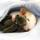There is no solution in integers unless the difference J – S is even. If J and S each received 5000 votes, then the difference is 0; if J received 5000 + X votes, then S must have received 5000 – X votes, so J – S = ( 5000 + X ) – ( 5000 – X ) = 2X.

ratboy (15167)“Great Answer” (0) Flag as…@ratboy speaks truth. I probably should have pointed that out last night, but I wanted you to get it yourself. Not everyone thinks through their problems when writing them, which means that not every problem is written well. This particular problem is not written well. You should show your work and then write an explanation of why the question does not make sense (why it doesn’t work).

bobbinhood (5896)“Great Answer” (0) Flag as…or